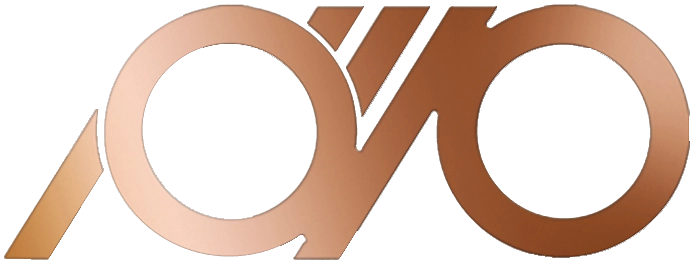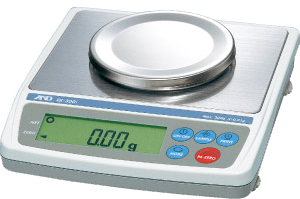## QAEM Copper Industries

Guide: To calculate the weight per meter of the tube, enter the outer diameter in millimeters in the OD section and the thickness in the WT section. Also, if you need to know the weight for any desired length, enter the desired length in millimeters in the L field. Finally, the calculation results will be visible in the Unit Weight (meter unit weight) and Weight (desired length weight) sections based on gram units.

Below you can calculate the unit weight and the total weight of an optional copper tube: